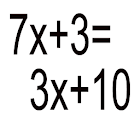All Android applications categories

All Android games categories# linear1

6 8.6

8.6 Users
rating

## Screenshots

Description

This app raises the algebra drill program to a whole new level. Instead of simply presenting the problem and leaving the student to work out the steps on paper, the student is lead through a succession of steps, with instant feedback at each step. In this manner the student can quickly identify and understand his/her errors. The student also avoids the tedium of writing out the intermediate equations corresponding to these steps and worrying about keeping the equations neat and having enough space on the paper. The instructions with this app also provide a nice tutorial on the subject.

This app deals with a simple type of algebra problem: one linear equation in one unknown. This type of equation is a first step in the solution of many word problems; it is also fundamental to learning the more advanced concepts of algebra. The program begins with a single equation with all the constants generated randomly (so that the student is unlikely to ever see the same problem twice). The form of the equation is fairly general, having one variable term (x multiplied by a constant) and one constant term on each side of the equation. The student is lead through a 3 step process to solve the equation, to obtain a single numeric value for the unknown variable, x. The steps are structured so that the student's entry at each step can be checked, and instant feedback is provided.

Each step is completed by entering a number using a virtual numeric keypad with large buttons to minimize error. If the number entered is correct the student progresses to the next step. If the number entered is wrong the student is notified and prompted to re-enter the number until it is correct.

The arithmetic for each of the 3 steps (subtraction, or dividing by 2, 4, or 5 to get decimal answers) is simple enough to be performed mentally, without resort to pencil and paper. Hence drill with this app will have the added benefit of increasing proficiency in the simple mental arithmetic.

The program usually runs through a specified number of problems, and the number of errors is tallied after the last problem. This is most useful in a teacher-student setting, where the number of errors at the end of the sequence can be used to evaluate the student's progress, sparing the teacher the tedium of grading the problems individually. The number of problems in the sequence can be specified by the user.

from 6 reviews

"Great"

8.6